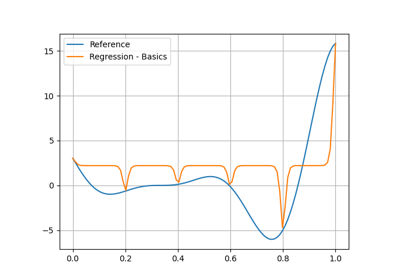gemseo / mlearning / regression

# gpr module¶

Gaussian process regression model.

## Overview¶

The Gaussian process regression (GPR) model expresses the model output as a weighted sum of kernel functions centered on the learning input data:

$y = \mu + w_1\kappa(\|x-x_1\|;\epsilon) + w_2\kappa(\|x-x_2\|;\epsilon) + ... + w_N\kappa(\|x-x_N\|;\epsilon)$

## Details¶

The GPR model relies on the assumption that the original model $$f$$ to replace is an instance of a Gaussian process (GP) with mean $$\mu$$ and covariance $$\sigma^2\kappa(\|x-x'\|;\epsilon)$$.

Then, the GP conditioned by the learning set $$(x_i,y_i)_{1\leq i \leq N}$$ is entirely defined by its expectation:

$\hat{f}(x) = \hat{\mu} + \hat{w}^T k(x)$

and its covariance:

$\hat{c}(x,x') = \hat{\sigma}^2 - k(x)^T K^{-1} k(x')$

where $$[\hat{\mu};\hat{w}]=([1_N~K]^T[1_N~K])^{-1}[1_N~K]^TY$$ with $$K_{ij}=\kappa(\|x_i-x_j\|;\hat{\epsilon})$$, $$k_i(x)=\kappa(\|x-x_i\|;\hat{\epsilon})$$ and $$Y_i=y_i$$.

The correlation length vector $$\epsilon$$ is estimated by numerical non-linear optimization.

## Surrogate model¶

The expectation $$\hat{f}$$ is the surrogate model of $$f$$.

## Error measure¶

The standard deviation $$\hat{s}$$ is a local error measure of $$\hat{f}$$:

$\hat{s}(x):=\sqrt{\hat{c}(x,x)}$

## Interpolation or regression¶

The GPR model can be regressive or interpolative according to the value of the nugget effect $$\alpha\geq 0$$ which is a regularization term applied to the correlation matrix $$K$$. When $$\alpha = 0$$, the surrogate model interpolates the learning data.

## Dependence¶

The GPR model relies on the GaussianProcessRegressor class of the scikit-learn library.

class gemseo.mlearning.regression.gpr.GaussianProcessRegressor(data, transformer=mappingproxy({}), input_names=None, output_names=None, kernel=None, bounds=None, alpha=1e-10, optimizer='fmin_l_bfgs_b', n_restarts_optimizer=10, random_state=None)[source]

Gaussian process regression model.

Parameters:
• data (IODataset) – The learning dataset.

• transformer (TransformerType) –

The strategies to transform the variables. The values are instances of Transformer while the keys are the names of either the variables or the groups of variables, e.g. "inputs" or "outputs" in the case of the regression algorithms. If a group is specified, the Transformer will be applied to all the variables of this group. If IDENTITY, do not transform the variables.

By default it is set to {}.

• input_names (Iterable[str] | None) – The names of the input variables. If None, consider all the input variables of the learning dataset.

• output_names (Iterable[str] | None) – The names of the output variables. If None, consider all the output variables of the learning dataset.

• kernel (Kernel | None) – The kernel specifying the covariance model. If None, use a Matérn(2.5).

• bounds (__Bounds | Mapping[str, __Bounds] | None) – The lower and upper bounds of the parameter length scales when kernel is None. Either a unique lower-upper pair common to all the inputs or lower-upper pairs for some of them. When bounds is None or when an input has no pair, the lower bound is 0.01 and the upper bound is 100.

• alpha (float | ndarray) –

The nugget effect to regularize the model.

By default it is set to 1e-10.

• optimizer (str | Callable) –

The optimization algorithm to find the parameter length scales.

By default it is set to “fmin_l_bfgs_b”.

• n_restarts_optimizer (int) –

The number of restarts of the optimizer.

By default it is set to 10.

• random_state (int | None) – The seed used to initialize the centers. If None, the random number generator is the RandomState instance used by numpy.random.

Raises:

ValueError – When both the variable and the group it belongs to have a transformer.

predict_std(input_data)[source]

Predict the standard deviation from input data.

The user can specify these input data either as a NumPy array, e.g. array([1., 2., 3.]) or as a dictionary of NumPy arrays, e.g. {'a': array([1.]), 'b': array([2., 3.])}.

If the NumPy arrays are of dimension 2, their i-th rows represent the input data of the i-th sample; while if the NumPy arrays are of dimension 1, there is a single sample.

Parameters:

input_data (ndarray | Mapping[str, ndarray]) – The input data.

Returns:

The standard deviation at the query points.

Return type:

ndarray

Warning

If the output variables are transformed before the training stage, then the standard deviation is related to this transformed output space unlike predict() which returns values in the original output space.

LIBRARY: Final[str] = 'scikit-learn'

The name of the library of the wrapped machine learning algorithm.

SHORT_ALGO_NAME: ClassVar[str] = 'GPR'

The short name of the machine learning algorithm, often an acronym.

Typically used for composite names, e.g. f"{algo.SHORT_ALGO_NAME}_{dataset.name}" or f"{algo.SHORT_ALGO_NAME}_{discipline.name}".

algo: Any

The interfaced machine learning algorithm.

input_names: list[str]

The names of the input variables.

input_space_center: dict[str, ndarray]

The center of the input space.

property kernel

The kernel used for prediction.

learning_set: Dataset

The learning dataset.

output_names: list[str]

The names of the output variables.

parameters: dict[str, MLAlgoParameterType]

The parameters of the machine learning algorithm.

transformer: dict[str, Transformer]

The strategies to transform the variables, if any.

The values are instances of Transformer while the keys are the names of either the variables or the groups of variables, e.g. “inputs” or “outputs” in the case of the regression algorithms. If a group is specified, the Transformer will be applied to all the variables of this group.

## Examples using GaussianProcessRegressor¶GP regression

GP regression